# 1. Birch and Swinnerton-Dyer's Hunch and Sato-Tate

## Sato-Tate for 11a (rank 0) for $p<10^9$## Sato-Tate for 5077a (rank 3) for $p<10^9$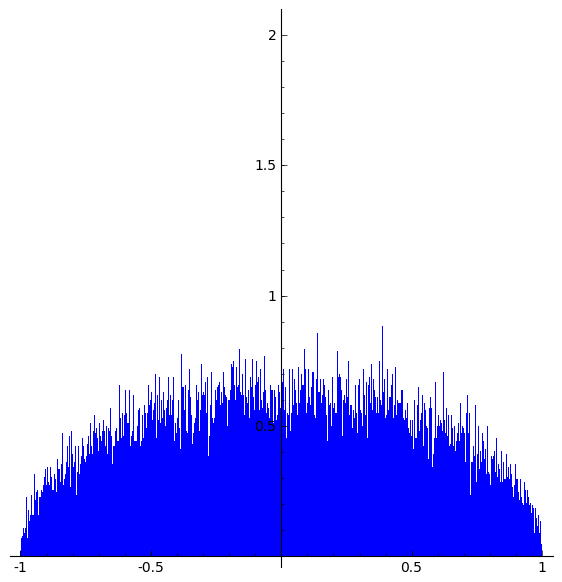# 2. Chebyshev Bias

## Distribution of primes mod 4 for $p < 10^9$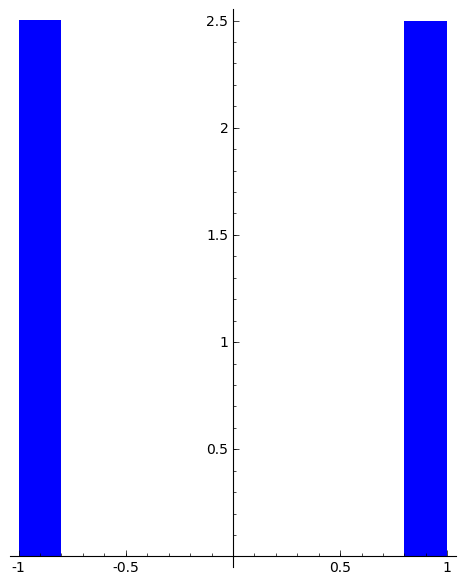## Prime race mod 4:   $\sum_{2 < p\leq X} \left(\frac{-1}{p}\right)$ for $p < 10^9$# 3. Riemann's Explicit Formula

## Oscillatory Term## $\varepsilon(X,T)$# 4. Raw, Medium, and Well Done Sums

## Raw Data on a Log Scale for 11a (rank 0) for $p<10^9$## Raw Data on a Log Scale for 5077a (rank 3) for $p<10^9$## Medium Data on a Log Scale for 11a (rank 0) for $p<10^9$## Medium Data on a Log Scale for 5077a (rank 3) for $p<10^9$## Well Done Data on a Log Scale for 11a (rank 0) for $p<10^9$## Well Done Data on a Log Scale for 5077a (rank 3) for $p<10^9$# 5. Sarnak's Letter - Conjectures

## Raw Mean on a Log Scale for 11a (rank 0) for $p<10^9$## Raw Mean on a Log Scale for 5077a (rank 3) for $p<10^9$## Medium Mean on a Log Scale for 11a (rank 0) for $p<10^9$## Medium Mean on a Log Scale for 5077a (rank 3) for $p<10^9$## Well Done Mean on a Log Scale for 11a (rank 0) for $p<10^9$## Well Done Mean on a Log Scale for 5077a (rank 3) for $p<10^9$# 6. Minimalist Conjecture?

## Raw Mean on a Log Scale for 128b (rank 0, but Sym^{7} extra vanishing) for $p<10^9$## Raw Mean on a Log Scale for 2379b (rank 0, but Sym^{3} extra vanishing) for $p<10^9$# 7. Oscillatory Term -- the Bayesian

## Plot of Oscillatory Term S(X,T)/log(X) for $X<10^{30}$ (log scale) and with $T$ changing from small to $500$ (animated) for 11a (rank 0)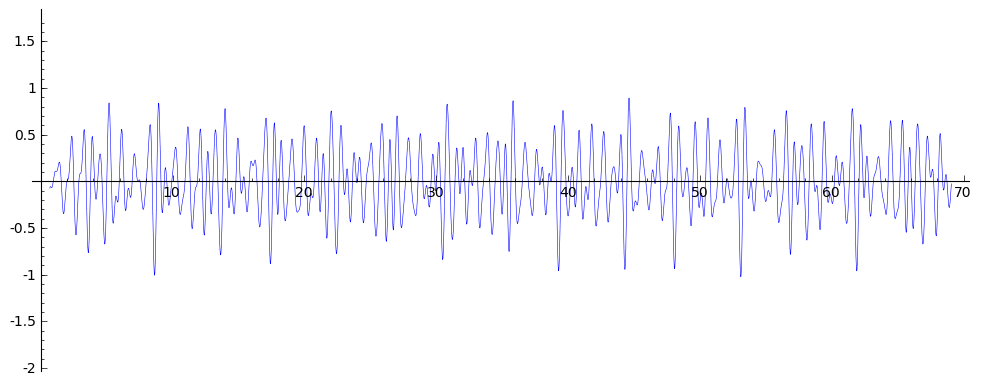## Plot of Oscillatory Term S(X,T)/log(X) for $X<10^{30}$ (log scale) and with $T$ changing from small to $500$ (animated) for 128b (rank 0, sym pow)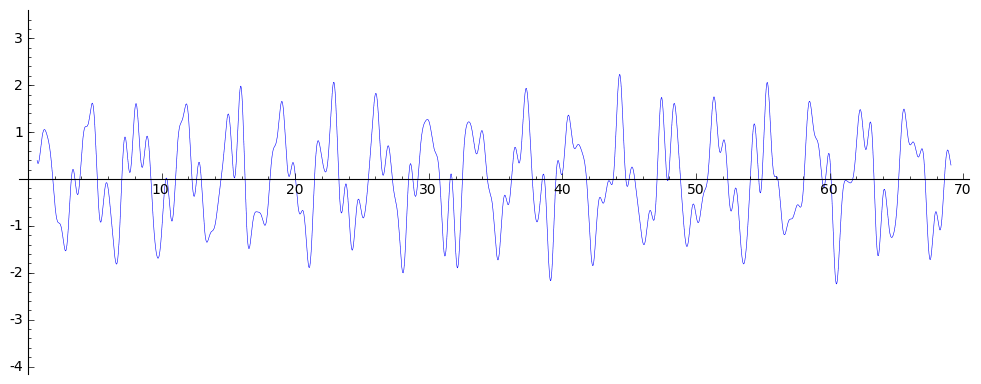## Plot of Oscillatory Term S(X,T)/log(X) for $X<10^{30}$ (log scale) and with $T$ changing from small to $500$ (animated) for 5077a (rank 3)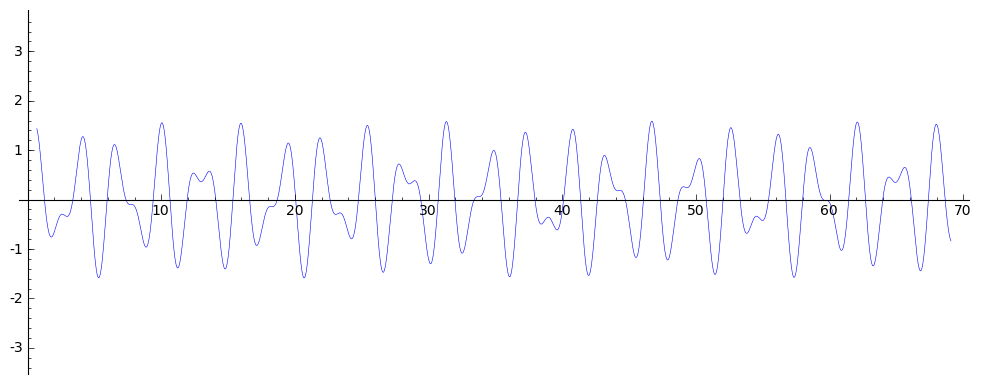## Plot of Distribution of Values of S(X,10000) for 11a (rank 0)## Plot of Distribution of Values of S(X,10000) for 128b (rank 0)## Plot of Distribution of Values of S(X,10000) for 5077a (rank 3)# 8. Convergent Term

## Plot of C(X,T) for 11a (rank 0) for $X<10^9$ and $T=10000$